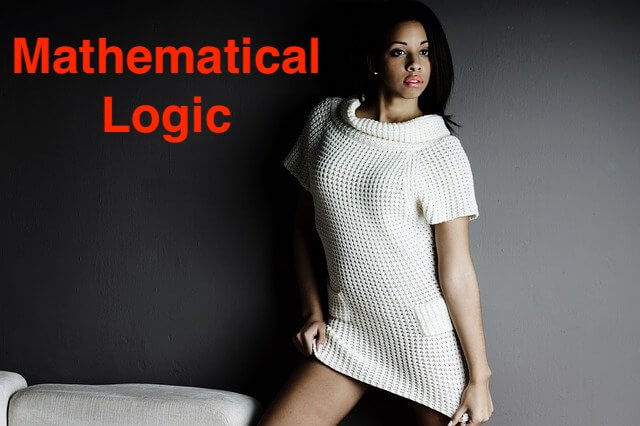Determine the truth value of the following statement. x + y = 0 is the equation of a straight line if and only if y2 = 4x is the equation of the parabola.

QUESTION

Miscellaneous Exercise 1 | Q 4.06 | Page 32

Determine the truth value of the following statement.

x + y = 0 is the equation of a straight line if and only if y2 = 4x is the equation of the parabola.

SOLUTION

Let p : x + y = 0 is the equation of a straight line.
q : y2 = 4x is the equation of the parabola.

The truth values of p and q are T and T respectively.
The given statement in symbolic form is p ↔ q.

∴ p ↔ q ≡ T ↔ T ≡ T

∴ Truth value of the given statement is T.